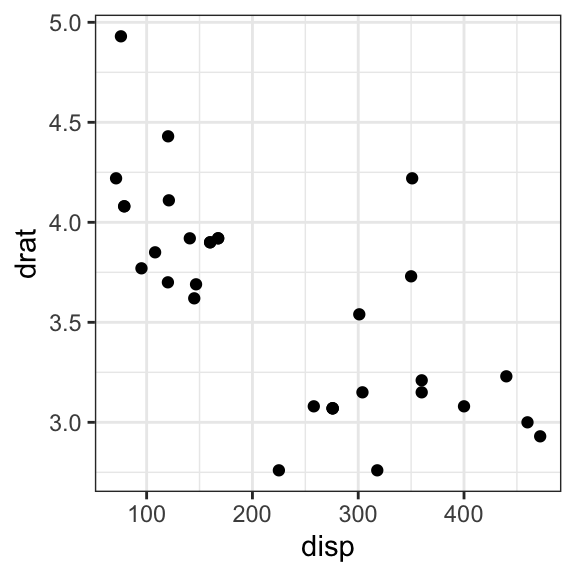# GGPlot AES: How to Assign Aesthetics in GGPlot2#### GGPlot AES: How to Assign Aesthetics in GGPlot2

In this article, you will learn how to map variables in the data to visual properpeties of ggplot geoms (points, bars, box plot, etc).

These visual caracteristics are known as aesthetics (or aes) and include:

• color and fill
• points shape
• line type
• size
• group
• etc

Aesthetic mappings can be defined in ggplot() and in individual layers (such as geom_point(), geom_line(), etc).

Contents:

#### Related Book

GGPlot2 Essentials for Great Data Visualization in R

## Prerequisites

Load required packages and set the theme function theme_bw() as the default theme:

library(ggplot2)
theme_set(theme_bw())

## Basics

Map aesthetics to variables and to functions of variables:

# Map aesthetics to variables
ggplot(ToothGrowth, aes(x = supp, y = len)) +
geom_boxplot()

# Map aesthetics to functions of variables
ggplot(mtcars, aes(x = mpg ^ 2, y = wt / cyl)) +
geom_point()Aesthetics can be also mapped to constants:

# map x to constant: 1
ggplot(ToothGrowth, aes(x = factor(1), y = len)) +
geom_boxplot(width = 0.5) +
geom_jitter(width = 0.1)Note that, aes() is passed to either ggplot() or to specific layer. Aesthetics specified to ggplot() are used as defaults for every layer.

For example:

# Use this
ggplot(mpg, aes(displ, hwy)) + geom_point()

# or this
ggplot(mpg) + geom_point(aes(displ, hwy))

## Color and fill

# Color
ggplot(ToothGrowth, aes(supp, len)) +
geom_boxplot(aes(color = supp))

# Fill
ggplot(ToothGrowth, aes(supp, len)) +
geom_boxplot(aes(fill = supp))## Shape

Change point shapes by groups:

ggplot(iris, aes(Sepal.Length, Sepal.Width)) +
geom_point(aes(shape = Species))## Group and line type

In line plot, for example, group aesthetic is used to ensure lines are drawn separately for each group

# Data
df2 <- data.frame(supp=rep(c("VC", "OJ"), each=3),
dose=rep(c("D0.5", "D1", "D2"),2),
len=c(6.8, 15, 33, 4.2, 10, 29.5))

head(df2, 4)
##   supp dose  len
## 1   VC D0.5  6.8
## 2   VC   D1 15.0
## 3   VC   D2 33.0
## 4   OJ D0.5  4.2
# Create a grouped line plot
ggplot(df2, aes(dose, len, group = supp)) +
geom_line() +
geom_point()

# Change linetype by groups
ggplot(df2, aes(dose, len, group = supp)) +
geom_line(aes(linetype = supp)) +
geom_point()## Label

ggplot(df2, aes(dose, len, group = supp)) +
geom_line() +
geom_point() +
geom_text(aes(label = len, vjust = -0.5))## Create wrappers around ggplot2 pipelines

aes() automatically quotes all its arguments, so you need to use tidy-evaluation to create wrappers around ggplot2 pipelines.

1. Simplest case: your wrapper takes dots
scatter_plot <- function(data, ...) {
ggplot(data) + geom_point(aes(...))
}
scatter_plot(mtcars, disp, drat)1. Your wrapper has named arguments. You need “enquote and unquote”
scatter_plot <- function(data, x, y) {
x <- enquo(x)
y <- enquo(y)

ggplot(data) + geom_point(aes(!!x, !!y))
}
scatter_plot(mtcars, disp, drat)Note that, users of your wrapper can use their own functions in the quoted expressions.

cut3 <- function(x) cut_number(x, 3)
scatter_plot(mtcars, cut3(disp), drat)